###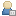Show Posts

This section allows you to view all posts made by this member. Note that you can only see posts made in areas you currently have access to.

### Topics - rain_storm

Pages: 1 2  4 5
41
##### Projects / rain by rain_storm
« on: June 03, 2008 »
here is the latest thing that Ive been working on and this time its only 64 bytes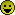I really hope that this works for most people anyone who isnt getting something like the image below could you use the screeny version to do a screen capture

42
##### ASM / mode 13h Screen capture routine
« on: June 03, 2008 »
This one took awhile to get around to doing but I finally knuckled down and had a look at the bmp format and this screen capture routine is the result it. This routine is ready to use out of the box just find the terminating ret byte (opcode 0xC3) from the end of your com file and replace it with the contents of SCREEN.BIN all offsets that are required are calculated within the routine. And its just 179 bytes in size.

43
« on: June 02, 2008 »
I just got the all clear for some webhosting over at untergrund.net but I dont have a clue how to actually upload intros there. I have managed to figure out how to open a ftp site in internet explorer but no matter what I try I cant login to my user account on the ftp site, my login works fine for the untergrund html site

Is there anyone here that has an untergrund account and can you explain to me what I need to do in simple words

44
##### General chat / The futer of 256 :(
« on: May 31, 2008 »
I was looking through some of the 256b intros on Pirx's website and came across the pdf file at the bottom of this page
http://66.102.9.104/translate_c?hl=en&sl=pl&u=http://ind.untergrund.net/&prev=/search%3Fq%3Dart_256b.pdf%26hl%3Den%26rlz%3D1T4GGLJ_enIE274IE274

To summarize it basically states how amazing pieces of art can be crammed into 256 bytes and with the onset of Vista this niche will become a thing of the past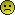I just cant shake the idea of the scene losing out on some fabulous tiny code as being a bad thing. I just dont want to see this die, I personnaly think that this category has become one of the least appreciated categories too. Just take a look at pouet and see what they have to say about 90% of all <256b demos. Its not good. But in order to get something good up and running in this category requires alot of experience about the target environment (in this case MS-DOS using a VGA display adapter), and more dirty tricks than the CIA and FBI combined.

So whats in store for the futer? Are we being forced to use DosBox... perish the thought cos DosBox is the worst way to view these demos. Perhaps 512b boot sectors will become the new 256b intros. this is even more niche than the current state of affairs since not many XP systems have Floppy drives and Vista is the same. still I think this is one possible direction. If boot sectors are the futer of tiny tiny code than Im gonna have to learn how to set a video mode by hand which may take as many as 256 bytes to achieve. I'll leave that until I have no other options. Thankfully there will still be places like 256b / intro inferno / hard code / pouet and of course dbfinteractive.

Anyone want to try cheering me up over this one

45
##### General coding questions / What makes Crinkler happy?
« on: May 26, 2008 »
Still playing around with this excellent tool but it just occured to me I havent a notion as to what to do to get the most out of crinkler. At the moment Im just trying something and linking with crinkler its not until i see the output that I can tell what difference any changes made, How about arrays do the compress well if they are not all zeros?

Edit:
Also what alternatives are there to crinkler Ive seen some demos packed in a com file that emits a MZ.exe whats that all about?

46
##### Challenges & Competitions / [procedural] fire
« on: May 12, 2008 »
had a damn hard time posting this one because of the picture size / format. kept getting the "you already submitted this post perhaps you double clicked post" message. but still no new topic created :/
anyways this is a 128 byte fire effect and my second entry into the compo. DOSBOX does not do a great job emulating this one you dont get the same colours as what I get when I run it through XP built in emulation. So only those with a video adapter that supports mode 13h will see the proper colours

47
##### ASM / Double Buffering
« on: May 07, 2008 »
syncing to the vertical retrace is not always enough to ensure that your frame will be drawn without slicing. If it takes you longer to draw a scan line than the strict timing allowed for you might want to try double buffering. It is a simple matter to add double buffering to a program without having to define the 64k buffer yourself.

Here is an example that double buffers and is suitable for com files up to 52992 bytes in size. This leaves you plenty of space for what not when making a 256b or even 1k/4k vga demos. The value 07D00h is the maximum starting address that works and is unused and will give you 52992 bytes for you executable. 07000h is the segment where your com file gets loaded into memory and you will be using some of this segment for the buffer.

Of course you should only use this if you have room to spare and your demo is experiencing slicing even with syncing enabled. The better thing to do is improve the speed of the drawing routine if possible. not always possible though (example drawing vertical lines / polygons)

Code: [Select]
` ORG 100h PALLET = 003C8h ; PALLET INDEX REGISTER VTRACE = 003DAh ; VERTICAL RETRACE STATUS PORT ADDRESS KEYBRD = 00060h ; KEYBOARD INPUT PORT ADDRESS VIDMEM = 0A000h ; VIDEO SEGMENT ADDRESS BUFFER = 07D00h ; DOUBLE BUFFER ADDRESS (MUST BE ABOVE 07010h FOR 256b / 07100h for 1k) VIDSIZ = 0FA00h ; SIZE OF SCREEN IN BYTES INIT:MOV   AX,13h      INT   10h      PUSH  VIDMEM      POP   ES      PUSH  BUFFER      POP   DS COLR:MOV   DX,PALLET      XOR   AX,AX      OUT   DX,AL      INC   DX GREY:OUT   DX,AL      OUT   DX,AL      OUT   DX,AL      INC   AX      JNZ   GREY MAIN:XOR   DI,DI      XOR   SI,SI      MOV   CX,VIDSIZ/4      MOV   DX,VTRACE SYNC:IN    AL,DX      TEST  AL,08h      JZ    SYNC      REP   MOVSD      XOR   SI,SI      MOV   CX,VIDSIZ/2 DRAW:MOV   AX,[DS:SI]      INC   AL      INC   AH      MOV   [DS:SI],AX      ADD   SI,2      LOOP  DRAW EXIT:IN    AL,KEYBRD      DEC   AL      JNZ   MAIN      RET`
EDIT:
I just wanted to add that double buffering in this manner has more than doubled the fps on any example ive thrown it into

48
##### ASM / VGA Floor Casting
« on: May 02, 2008 »
I really wanted to enter this into the procedural compo but since its based on a previous work by me I think its best not to, anyways this is my very first 256 byte intro hope you all like it source and com file included (assembled with FASM)

49
##### Challenges & Competitions / [PROCEDURAL] TUNNEL
« on: April 20, 2008 »
Here's my entry into the procedural compo, It may require DosBox for those of you with Vista.
I have used a circle drawing routine for this that I obtained here

Source and Exe included.

50
##### Yabasic / ps2Yabasic faster than win32Yabasic
« on: April 04, 2008 »
I have just tested code on both Jims emulator and on the win32 emulator and am pleased to announce that Jims version runs the same code alot faster and smoother. But the win32 emulator has some problems with user input so its not the exact same file. But that is only done once per frame so it aint that part that is slowing it all down. Dont need to test on ps2 cos we all know that it will run slower there.

51
##### ASM / OpenGL Example Full Screen
« on: April 03, 2008 »
This is the openGL example that comes with FASM but I changed it so that it ran in full screen instead of a window. Hope someone finds this useful

Code: [Select]
`; OpenGL programming exampleformat PE GUI 4.0entry startinclude 'win32a.inc'include 'opengl.inc'RESX = 640RESY = 480FULLSCREEN = WS_VISIBLE+WS_MAXIMIZE+WS_POPUPWINDOW = WS_VISIBLE+WS_OVERLAPPEDWINDOW+WS_CLIPCHILDREN+WS_CLIPSIBLINGSsection '.data' data readable writeable  _title db 'OPENGL FULLSCREEN',0  _class db 'OPENGL',0  wc WNDCLASS 0,WindowProc,0,0,NULL,NULL,NULL,NULL,NULL,_class  hwnd dd ?  hdc dd ?  hrc dd ?  msg MSG  rc RECT  pfd PIXELFORMATDESCRIPTOR  active dd ?section '.code' code readable executable  start: invoke GetModuleHandle,0 mov [wc.hInstance],eax invoke LoadIcon,0,IDI_APPLICATION mov [wc.hIcon],eax invoke LoadCursor,0,IDC_ARROW mov [wc.hCursor],eax invoke RegisterClass,wc ;invoke  CreateWindowEx,0,_class,_title,WINDOW,32,32,RESX,RESY,NULL,NULL,[wc.hInstance],NULL invoke CreateWindowEx,NULL,_class,_title,FULLSCREEN,32,32,RESX,RESY,NULL,NULL,[wc.hInstance],NULL mov [hwnd],eax  msg_loop: invoke InvalidateRect,[hwnd],NULL,FALSE invoke GetMessage,msg,NULL,0,0 or eax,eax jz end_loop invoke TranslateMessage,msg invoke DispatchMessage,msg jmp msg_loop  end_loop: invoke ExitProcess,[msg.wParam]proc WindowProc hwnd,wmsg,wparam,lparam push ebx esi edi cmp [wmsg],WM_CREATE je .wmcreate cmp [wmsg],WM_SIZE je .wmsize cmp [wmsg],WM_ACTIVATEAPP je .wmactivateapp cmp [wmsg],WM_PAINT je .wmpaint cmp [wmsg],WM_KEYDOWN je .wmkeydown cmp [wmsg],WM_DESTROY je .wmdestroy  .defwndproc: invoke DefWindowProc,[hwnd],[wmsg],[wparam],[lparam] jmp .finish  .wmcreate: invoke GetDC,[hwnd] mov [hdc],eax mov edi,pfd mov ecx,sizeof.PIXELFORMATDESCRIPTOR shr 2 xor eax,eax rep stosd mov [pfd.nSize],sizeof.PIXELFORMATDESCRIPTOR mov [pfd.nVersion],1 mov [pfd.dwFlags],PFD_SUPPORT_OPENGL+PFD_DOUBLEBUFFER+PFD_DRAW_TO_WINDOW mov [pfd.dwLayerMask],PFD_MAIN_PLANE mov [pfd.iPixelType],PFD_TYPE_RGBA mov [pfd.cColorBits],32 mov [pfd.cDepthBits],32 mov [pfd.cAccumBits],0 mov [pfd.cStencilBits],0 invoke ChoosePixelFormat,[hdc],pfd invoke SetPixelFormat,[hdc],eax,pfd invoke wglCreateContext,[hdc] mov [hrc],eax invoke wglMakeCurrent,[hdc],[hrc] invoke GetClientRect,[hwnd],rc invoke glViewport,0,0,[rc.right],[rc.bottom] xor eax,eax jmp .finish  .wmsize: invoke GetClientRect,[hwnd],rc invoke glViewport,0,0,[rc.right],[rc.bottom] invoke InvalidateRect,[hwnd],NULL,FALSE xor eax,eax jmp .finish  .wmactivateapp: push [wmsg] pop [active] xor eax,eax jmp .finish  .wmpaint: invoke glClear,GL_COLOR_BUFFER_BIT invoke glRotatef, 1.0, 0.0, 0.0, 1.0 invoke glBegin,GL_QUADS invoke glColor3f,  0.0, 1.0, 1.0 invoke glVertex3f,-0.5,-0.5,-0.5 invoke glColor3f,  1.0, 0.0, 1.0 invoke glVertex3f, 0.5,-0.5,-0.5 invoke glColor3f,  1.0, 1.0, 0.0 invoke glVertex3f, 0.5, 0.5,-0.5 invoke glColor3f,  0.0, 0.0, 1.0 invoke glVertex3f,-0.5, 0.5,-0.5 invoke glEnd invoke SwapBuffers,[hdc] xor eax,eax jmp .finish  .wmkeydown: cmp [wparam],VK_ESCAPE jne .defwndproc  .wmdestroy: invoke wglMakeCurrent,0,0 invoke wglDeleteContext,[hrc] invoke ReleaseDC,[hwnd],[hdc] invoke PostQuitMessage,0 xor eax,eax  .finish: pop edi esi ebx retendpsection '.idata' import data readable writeable  library kernel,'KERNEL32.DLL',\   user,'USER32.DLL',\   gdi,'GDI32.DLL',\   opengl,'OPENGL32.DLL'  import kernel,\ GetModuleHandle,'GetModuleHandleA',\ ExitProcess,'ExitProcess'  import user,\ RegisterClass,'RegisterClassA',\ CreateWindowEx,'CreateWindowExA',\ DefWindowProc,'DefWindowProcA',\ GetMessage,'GetMessageA',\ TranslateMessage,'TranslateMessage',\ DispatchMessage,'DispatchMessageA',\ LoadCursor,'LoadCursorA',\ LoadIcon,'LoadIconA',\ GetClientRect,'GetClientRect',\ InvalidateRect,'InvalidateRect',\ GetDC,'GetDC',\ ReleaseDC,'ReleaseDC',\ PostQuitMessage,'PostQuitMessage'  import gdi,\ ChoosePixelFormat,'ChoosePixelFormat',\ SetPixelFormat,'SetPixelFormat',\ SwapBuffers,'SwapBuffers'  import opengl,\ wglCreateContext,'wglCreateContext',\ wglMakeCurrent,'wglMakeCurrent',\ glViewport,'glViewport',\ glClear,'glClear',\ glRotatef,'glRotatef',\ glBegin,'glBegin',\ glColor3f,'glColor3f',\ glVertex3f,'glVertex3f',\ glEnd,'glEnd',\ wglDeleteContext,'wglDeleteContext'`

52
##### General chat / Happy Birthday Hotshot
« on: March 03, 2008 »
Have a happy birthday buddy. Best wishes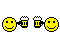53
##### Yabasic / Pathfinding in Yabasic
« on: February 10, 2008 »
This program uses tha A* pathfinding algorithm to find the shortest path between two points. It does find the shortest path but I think the code for adding new nodes to the open list and the code for finding the open node with the lowest cost could be improved apon a lot. Anyway here it goes :
Code: [Select]
`open window 640, 512gridX = 19gridY = 15dim grid(gridX, gridY)dim list(gridX, gridY)for y = 0 to gridY  for x = 0 to gridX    read grid(x, y)  nextnextposX = 2posY = 2tarX = 17tarY = 13label main  astar(posX, posY, tarX, tarY)  goto mainsub astar(px, py, tx, ty)  nodes = 1  closed = 0  for y = 0 to gridY    for x = 0 to gridX      list(x,y) = 0    next  next  dim nodeX(nodes), nodeY(nodes)  dim homeX(nodes), homeY(nodes)  dim cost(nodes)  nodeX(nodes) = px  nodeY(nodes) = py  homeX(nodes) = px  homeY(nodes) = py  cost(nodes) = abs(px-tx) + abs(py-ty)  list(px,py) = 1  cx = px  cy = py  while (closed < nodes)    render()    cost = (gridX+1)*(gridY+1)*10    for n = 1 to nodes      if (list(nodeX(n), nodeY(n)) = 1) and (cost(n) < cost) then        cost = cost(n)        cur = n      endif    next    cx = nodeX(cur)    cy = nodeY(cur)    cost = cost - (abs(tx-cx)+abs(ty-cy))    list (cx,cy) = 2    closed = closed + 1    for y = cy-1 to cy+1      for x = cx-1 to cx+1        if (grid(x, y) = 0) and (list(x, y) = 0) then          nodes = nodes + 1          redim nodeX(nodes), nodeY(nodes)          redim homeX(nodes), homeY(nodes)          redim cost(nodes)          nodeX(nodes) = x          nodeY(nodes) = y          homeX(nodes) = cx          homeY(nodes) = cy          if (abs(x-cx) = 1) and (abs(y-cy) = 1) then            cost(nodes) = cost + 14 + abs(tx-x) + abs(ty-y)          else            cost(nodes) = cost + 10 + abs(tx-x) + abs(ty-y)          endif          list(x,y) = 1        endif      next    next    if (list(tx,ty) <> 0) goto trace  wendlabel trace  while (inkey\$(0) = "")    render()    x = tx    y = ty    length = 0    for n = 1 to nodes      if (nodeX(n) = x) and (nodeY(n) = y) then        length = length + 1        redim pathX(length), pathY(length)        setrgb 1, 255, 255, 000        fill box x*s+8, y*s+8 to x*s+s-8, y*s+s-8        setrgb 1, 000, 000, 000        text x*s+16, y*s+10, str\$(length), "cc"        x = homeX(n)        y = homeY(n)        pathY(length) = y        pathX(length) = x        if (x <> nodeX(n)) or (y <> nodeY(n)) n = 1      endif    next  wendend subsub render()  setdispbuf draw  draw = 1 - draw  setdrawbuf draw  clear window  s = 32  for y = 0 to gridY    for x = 0 to gridX      setrgb 1, 010, 010, 010      if (grid(x, y) = 1) setrgb 1, 000, 255, 000      if (list(x, y) = 1) setrgb 1, 000, 000, 100      if (list(x, y) = 2) setrgb 1, 100, 000, 000      fill box x*s, y*s to x*s+s, y*s+s    next  next  setrgb 1, 100, 100, 100  for y = 0 to gridY    for x = 0 to gridX box x*s, y*s to x*s+s, y*s+s next  next  setrgb 1, 000, 000, 255  fill box posX*s, posY*s to posX*s+s, posY*s+s  setrgb 1, 255, 000, 000  fill box tarX*s, tarY*s to tarX*s+s, tarY*s+send subdata 1,1,1,1,1,1,1,1,1,1,1,1,1,1,1,1,1,1,1,1data 1,0,0,0,0,0,0,0,0,0,0,0,0,0,0,0,0,0,0,1data 1,0,0,0,1,1,1,1,1,0,1,0,1,1,1,1,1,1,0,1data 1,0,0,0,1,0,0,0,0,0,1,0,1,0,0,0,0,0,0,1data 1,0,1,1,1,0,1,1,1,1,1,0,1,0,1,1,1,1,1,1data 1,0,1,0,0,0,0,0,0,0,0,0,1,0,0,0,0,0,0,1data 1,0,1,0,1,1,1,1,1,0,1,0,1,1,1,1,1,1,0,1data 1,0,1,0,1,0,0,0,1,0,1,0,0,0,0,0,0,0,0,1data 1,0,1,0,1,0,1,0,1,0,1,0,1,1,1,1,1,1,0,1data 1,0,1,0,1,0,1,0,1,0,1,0,0,0,1,0,0,0,0,1data 1,0,1,0,1,0,1,0,1,0,1,0,1,0,1,0,1,1,1,1data 1,0,1,0,1,0,1,0,1,0,1,0,1,0,1,0,0,0,0,1data 1,0,1,0,1,0,1,0,1,0,1,0,1,0,1,0,0,0,0,1data 1,0,1,0,1,0,1,0,1,0,1,1,1,0,1,0,0,0,0,1data 1,0,0,0,0,0,1,0,0,0,0,0,0,0,0,0,0,0,0,1data 1,1,1,1,1,1,1,1,1,1,1,1,1,1,1,1,1,1,1,1`

54
##### General coding questions / Need help stabalizing collision physics
« on: January 31, 2008 »
How do I determine whether two 2D circles are colliding or just in contact with each other? The thing is that my circle - circle collisions are unstable when there are many circle all close together. Such that when two circles should be just barely touching each other (without exchanging momentum) but instead their are constantly in collision mode and freely exchange what little momentum is left. When there are layers of balls resting on top of other balls (so that some balls are not touching a solid surface beyond the surfaces of its neighbouring circles) some of these balls will still be under the effect of gravity which adds feul to the fire and causes all the circles to vibrate like a bunch of jitter bugs.

Now how do I differentiate between colliding with an object and merely resting whilst in contact with it. Any ideas are appreciated.

Heres the collision routine as it is now. To run it you will need Jims ps2Yabasic emulator which can be found in the first post of this topic: http://dbfinteractive.com/index.php?topic=758.0
Code: [Select]
`open window 640, 512tolerence = 1.10gravity   = 0.20friction  = 0.80bounce    = 0.80minmotion = 0.10move      = 0.0balls = 26dim posx(balls), posy(balls)dim dirx(balls), diry(balls)dim radi(balls)label reset  for b = 0 to balls    dirx(b) = ran(move) - ran(move)    diry(b) = ran(move) - ran(move)    radi(b) = 32 // int(ran(8)) + 160:  posx(b) = ran(640-radi(b)-radi(b)) + radi(b)    posy(b) = ran(512-radi(b)-radi(b)) + radi(b)    for a = 0 to b      if (a <> b) then        adj = abs(posx(a) - posx(b))        opp = abs(posy(a) - posy(b))        hyp = adj*adj + opp*opp        if (hyp <= (radi(a)+radi(b))*(radi(a)+radi(b))) goto 0      endif    next  nextwait 0.1label main  wait 0.01  setdispbuf draw  draw = 1 - draw  setdrawbuf draw  clear window  setrgb 1, 016, 006, 006  setrgb 2, 016, 006, 006  setrgb 3, 255, 100, 100  gtriangle 0,0 to 640,0 to 640,512  setrgb 2, 255, 100, 100  gtriangle 0,0 to 0,512 to 640,512  for b = 0 to balls    setrgb 1, 016, 016, 128    fill circle posx(b), posy(b), radi(b) * tolerence    clear fill circle posx(b), posy(b), radi(b)    rgb = 20    for r = radi(b) to 8 step - 4      setrgb 1, 000, 000, rgb      fill circle posx(b), posy(b), r      rgb = rgb + 20    next    diry(b) = diry(b) + gravity   next  if (inkey\$(0) <> "") then    goto reset  endif  for a = 0 to balls    if (dirx(a) <> 0.0) or (diry(a) <> 0.0) then    collision = 0    for b = a to balls    if (dirx(b) <> 0.0) or (diry(b) <> 0.0) then      if (a <> b) then        adj = posx(b) - posx(a)        opp = posy(b) - posy(a)        dist = adj^2 + opp^2        if (dist < (radi(a)+radi(b))*(radi(a)+radi(b))) then          dist = sqrt(dist)          nx = adj / dist          ny = opp / dist          cx = (posx(a) + posx(b))*0.5          cy = (posy(a) + posy(b))*0.5          posx(a) = cx - nx*radi(a)*tolerence          posy(a) = cy - ny*radi(a)*tolerence          posx(b) = cx + nx*radi(b)*tolerence          posy(b) = cy + ny*radi(b)*tolerence          product = (dirx(b)-dirx(a))*nx + (diry(b)-diry(a))*ny          dirx(a) = dirx(a) + nx * product * friction          diry(a) = diry(a) + ny * product * bounce          dirx(b) = dirx(b) - nx * product * friction          diry(b) = diry(b) - ny * product * bounce          if (posx(b) < radi(b)) posx(b) = radi(b)          if (posx(b) = radi(b)) dirx(b) =-dirx(b) * friction          if (posx(b) > 640-radi(b)) posx(b) = 640 - radi(b)          if (posx(b) = 640-radi(b)) dirx(b) =-dirx(b) * friction          if (posy(b) < radi(b)) posy(b) = radi(b)          if (posy(b) = radi(b)) diry(b) =-diry(b) * bounce          if (posy(b) > 512-radi(b)) posy(b) = 512-radi(b)          if (posy(b) = 512-radi(b)) diry(b) =-diry(b) * bounce          if (posx(a) < radi(a)) posx(a) = radi(a)          if (posx(a) = radi(a)) dirx(a) =-dirx(a) * friction          if (posx(a) > 640-radi(a)) posx(a) = 640 - radi(a)          if (posx(a) = 640-radi(a)) dirx(a) =-dirx(a) * friction          if (posy(a) < radi(a)) posy(a) = radi(a)          if (posy(a) = radi(a)) diry(a) =-diry(a) * bounce          if (posy(a) > 512-radi(a)) posy(a) = 512-radi(a)          if (posy(a) = 512-radi(a)) diry(a) =-diry(a) * bounce          collision = 1        fi      fi      endif    next    if (collision = 0) then      posx(a) = posx(a) + dirx(a)      posy(a) = posy(a) + diry(a)      if    (posx(a) < radi(a)) then        posx(a) = radi(a)        dirx(a) =-dirx(a) * friction      elsif (posx(a) > 640-radi(a)) then        posx(a) = 640 - radi(a)        dirx(a) =-dirx(a) * friction      fi      if    (posy(a) < radi(a)) then        posy(a) = radi(a)        diry(a) =-diry(a) * bounce      elsif (posy(a) > 512-radi(a)) then        posy(a) = 512-radi(a)        diry(a) =-diry(a) * bounce      fi    else      if (posx(a) < radi(a)) posx(a) = radi(a)      if (posx(a) = radi(a)) dirx(a) =-dirx(a) * friction      if (posx(a) > 640-radi(a)) posx(a) = 640 - radi(a)      if (posx(a) = 640-radi(a)) dirx(a) =-dirx(a) * friction      if (posy(a) < radi(a)) posy(a) = radi(a)      if (posy(a) = radi(a)) diry(a) =-diry(a) * bounce      if (posy(a) > 512-radi(a)) posy(a) = 512-radi(a)      if (posy(a) = 512-radi(a)) diry(a) =-diry(a) * bounce    endif    if (diry(a) >=-minmotion) and (diry(a) <= minmotion) then      diry(a) = 0.0    endif    if (dirx(a) >=-minmotion) and (dirx(a) <= minmotion) then      dirx(a) = 0.0    endif    endif  next  goto main`

55
##### Freebasic / Floor Casting With Xor Texture
« on: January 17, 2008 »
28 lines of code (not including white spaces or comments) fast and interesting. Not a bad effort if I say myself

Code: [Select]
`' Floor Castingconst resX = 640const resY = 480const xres = 320const yres = 240#include once "tinyptc.bi"if (ptc_open("FLOOR CASTING", resX, resY) = 0) then end -1dim shared as integer buffer(resX*resY)dim as single u, v, w, camX, camYdim as integer c, bwhile (inkey\$ = "")  camY += 1  camX = xres*sin(camY*3.14159/180)  b = 0  for y = 1 to resY    w  = -yres / y    v =  resX*w - camY    u = -xres*w + camX    for x = 1 to resX      c = (u xor v) and 255      buffer(b+x) = rgb(c,c,c)      u = u + w    next    b = b + resX  next  ptc_update@buffer(1)wendptc_closeend 0`

56
##### General chat / Happy Birthday Ferris
« on: December 27, 2007 »Happy bday dude

Hope ya have a good one

57
##### General chat / Happy Thanksgiving
« on: November 22, 2007 »
Im celebrating here in LA and man its good to be reunited with my cousins. I hope all the american members of this forum have a good time.58
##### General chat / Happy Birthday nystep
« on: November 11, 2007 »
Happy Birthday buddy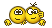Hope you have a great one59
##### Yabasic / Circle Line Segment Collision Detection & Response
« on: November 04, 2007 »
Here is a nice little routine that will allow for circles of any radius length and moving at any speed to collide with a finite line segment. Not only does this routine detect the collision it goes one further and repositions the circle so that it just touches the line segment. Use it freely, I got my inspiration for this routine from the following tutorial, I didn't just cut and paste it's my own interpretation of the solution.

http://www.harveycartel.org/metanet/tutorials/tutorialA.html

I'm very happy with how it works but I want to optimise it to the maximum and was wondering if anybody can figure out a way to remove the square roots. I know that this can be done easily within if statements by simply squaring the other value in the comparison ex:
Code: [Select]
`if (b   > sqrt(a))  rem this is equivelent to belowif (b*b > a)        rem this is equivelent to above`Can this trick be done to the values themselves? I cant seem to get it to work. Any ways here is the code for anyone who is interested

Edit :
Code: [Select]
`  bx = x2 - x1  by = y2 - y1  B = sqrt(bx*bx + by*by)  cs = r * by / B  sn = r * bx / B`Thats the square root I'm trying to eliminate. would it be possible if I squared bx,by then divided by B without a square root in sight

60
##### Challenges & Competitions / [Halloween]: Happy Halloween dbf :D
« on: October 24, 2007 »
Well here is my entry for the compo it runs in the ps2Yabasic emulator (Jims emulator) get it here:

http://members.iinet.net.au/~jimshaw/Yabasic/ps2yabasic1.6b3.zip

Pages: 1 2  4 5